## A ball rolling across the floor at a velocity of 6.15 m/s [E] slows to rest in 1.00 minute. The acceleration of the ball is m /s[E]. R

Question

A ball rolling across the floor at a velocity of 6.15 m/s [E] slows to rest in 1.00 minute. The
acceleration of the ball is m /s[E]. Record your answer to 3 significant digits.

in progress 0
1 month 2021-08-12T13:14:44+00:00 1 Answers 0 views 0

-0.103 m/s2

Explanation:

Acceleration is defined as the rate of change of speed per unit time. The change of speed is given by deducting the initial speed from the final speed and represented by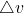Therefore, acceleration, a is given as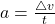where t is time in seconds.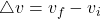where v represent velocity and subscripta f and i represent final and initial respectively. The formula for acceleration can then be re-written as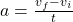Since the rolling ball finally comes to rest then the final velocity is 0. The time, 1 min is equal to 60 s.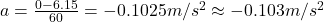The negative denote that it is deceleration